# Image enhancement

7 de Jan de 2014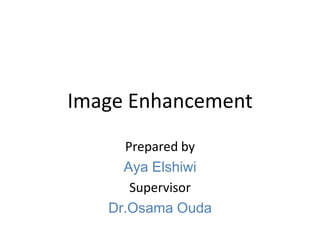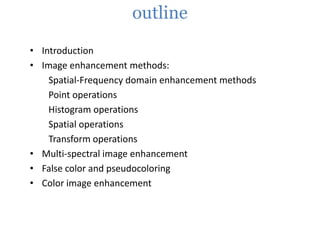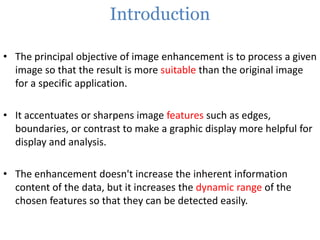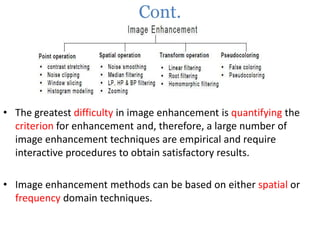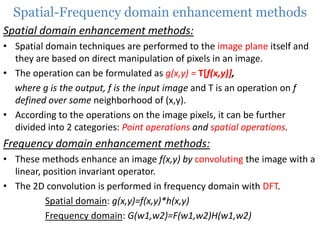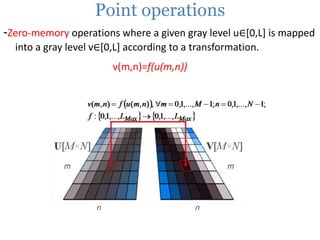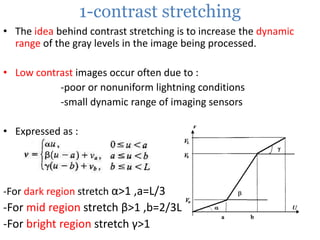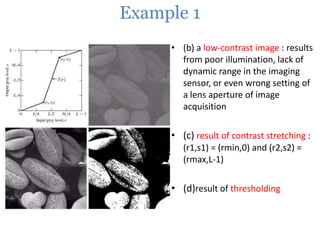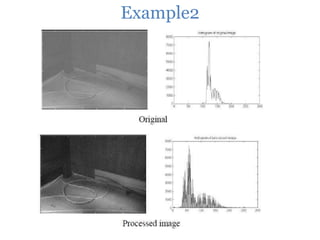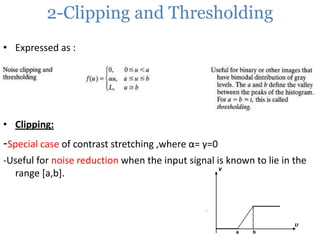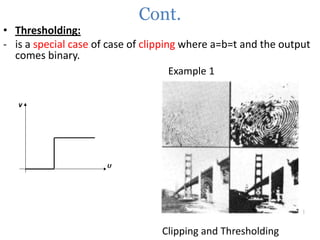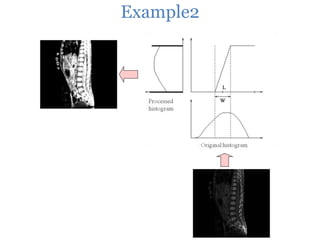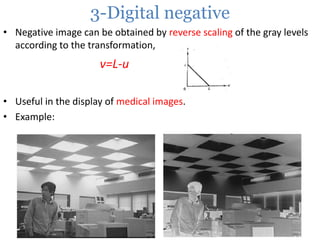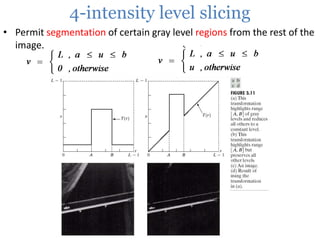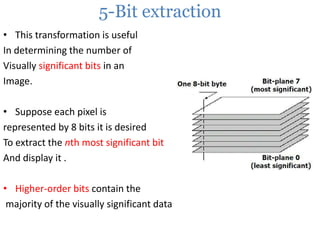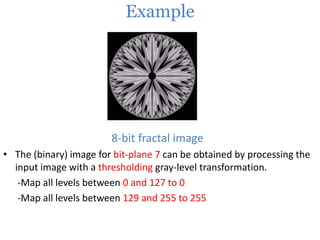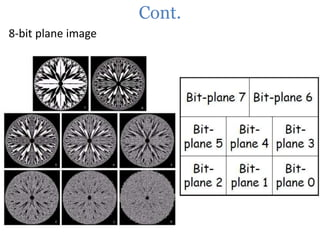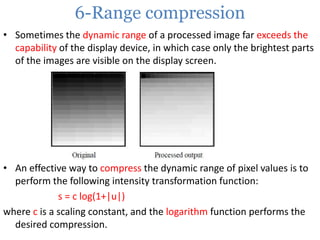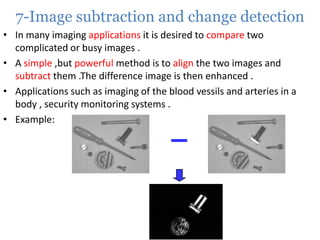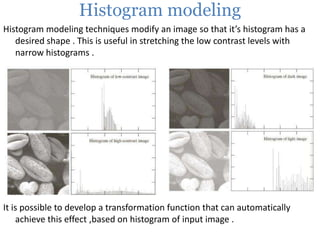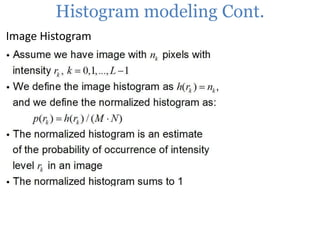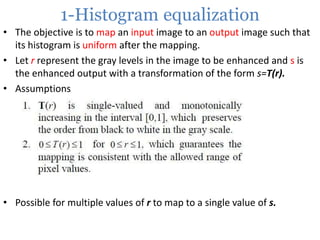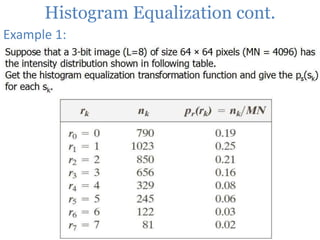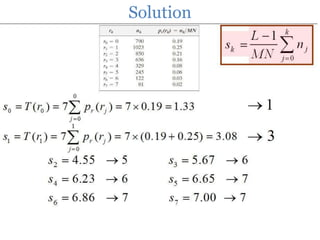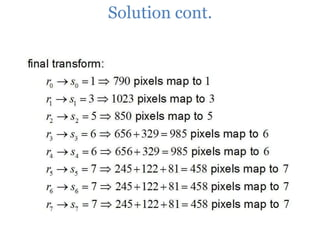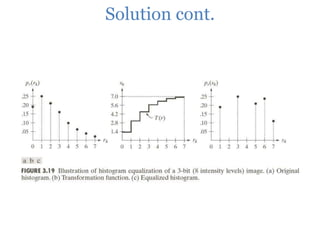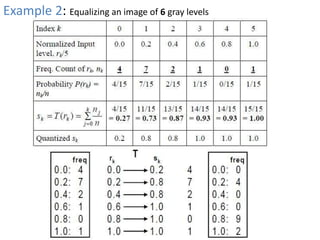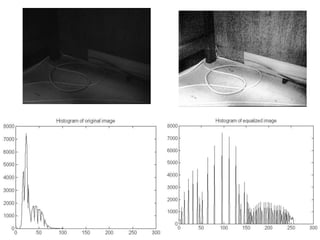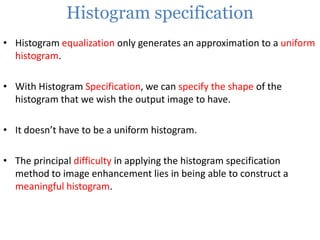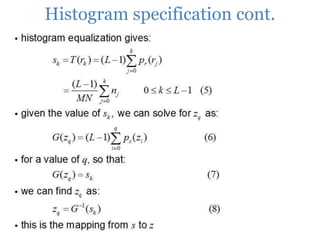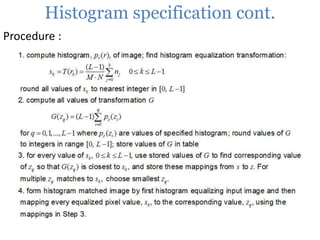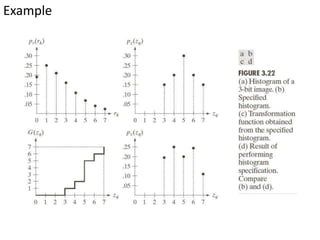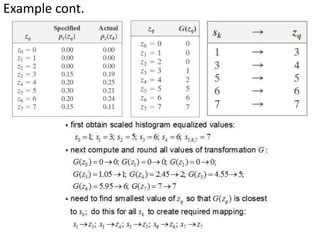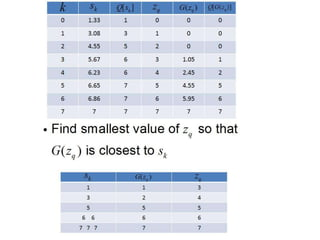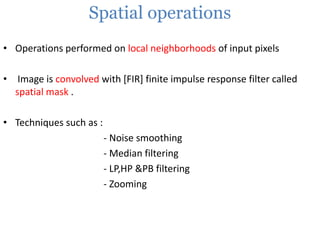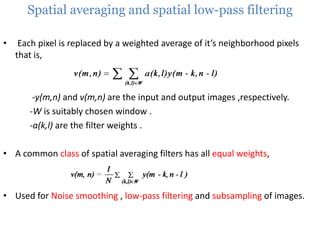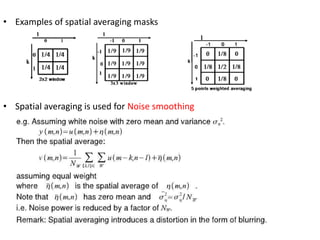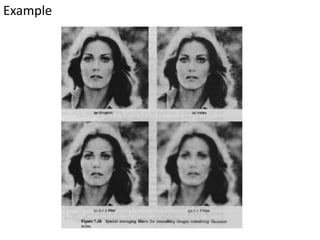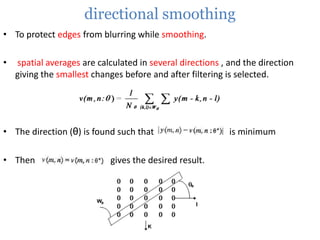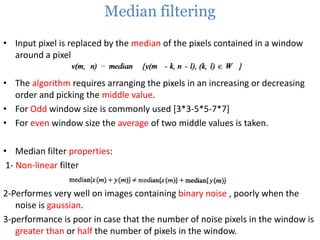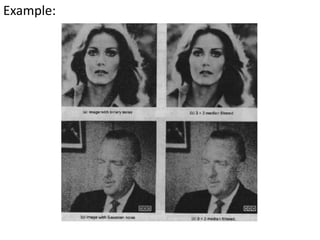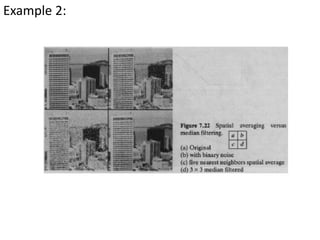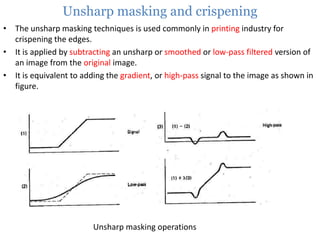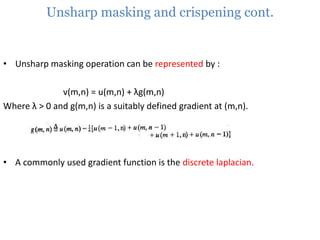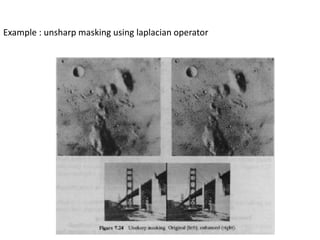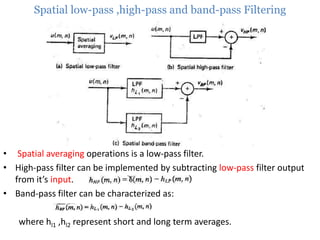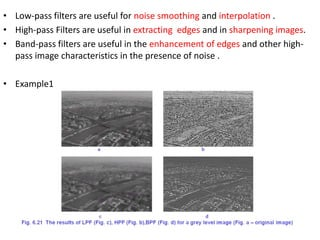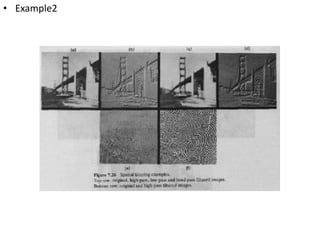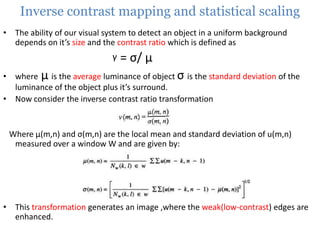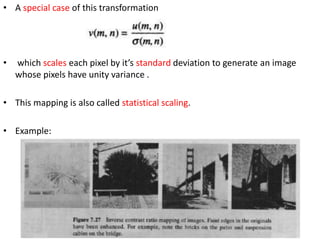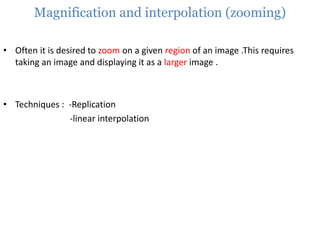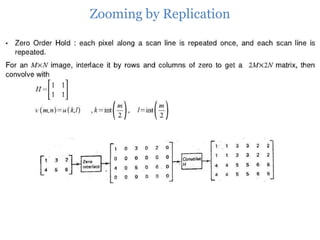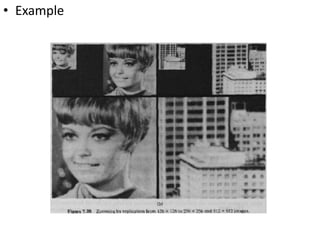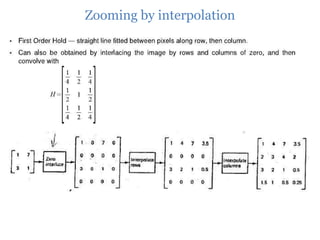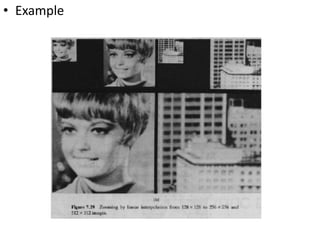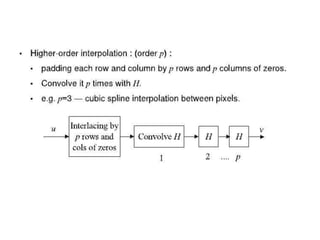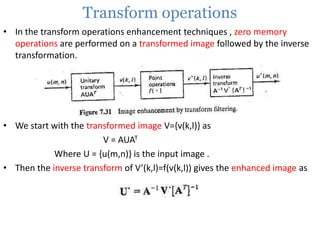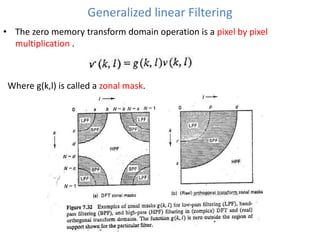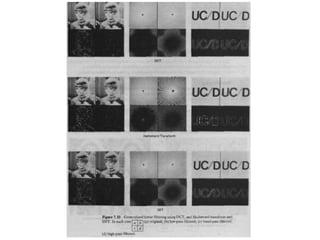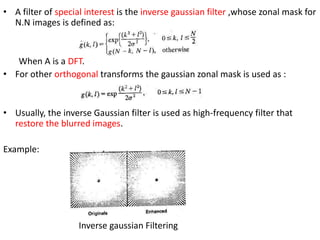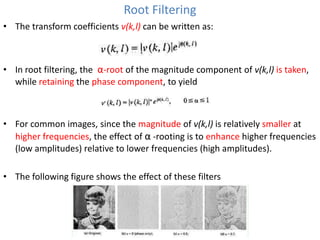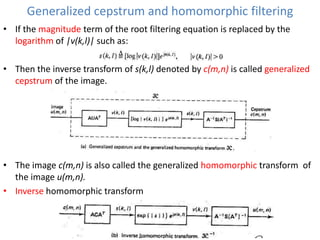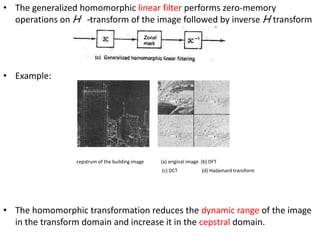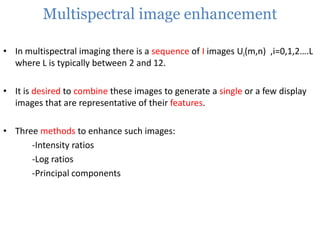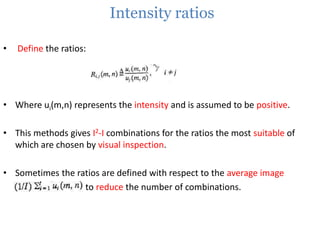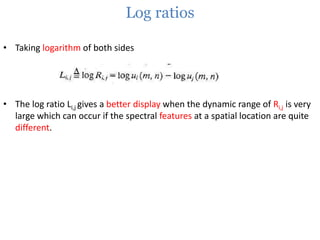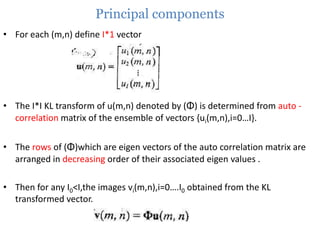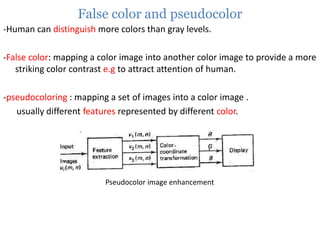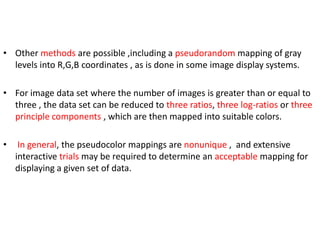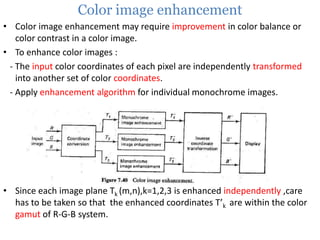1 de 71

### Image enhancement

• 1. Image Enhancement Prepared by Aya Elshiwi Supervisor Dr.Osama Ouda
• 2. outline • Introduction • Image enhancement methods: Spatial-Frequency domain enhancement methods Point operations Histogram operations Spatial operations Transform operations • Multi-spectral image enhancement • False color and pseudocoloring • Color image enhancement
• 3. Introduction • The principal objective of image enhancement is to process a given image so that the result is more suitable than the original image for a specific application. • It accentuates or sharpens image features such as edges, boundaries, or contrast to make a graphic display more helpful for display and analysis. • The enhancement doesn't increase the inherent information content of the data, but it increases the dynamic range of the chosen features so that they can be detected easily.
• 4. Cont. • The greatest difficulty in image enhancement is quantifying the criterion for enhancement and, therefore, a large number of image enhancement techniques are empirical and require interactive procedures to obtain satisfactory results. • Image enhancement methods can be based on either spatial or frequency domain techniques.
• 5. Spatial-Frequency domain enhancement methods Spatial domain enhancement methods: • Spatial domain techniques are performed to the image plane itself and they are based on direct manipulation of pixels in an image. • The operation can be formulated as g(x,y) = T[f(x,y)], where g is the output, f is the input image and T is an operation on f defined over some neighborhood of (x,y). • According to the operations on the image pixels, it can be further divided into 2 categories: Point operations and spatial operations. Frequency domain enhancement methods: • These methods enhance an image f(x,y) by convoluting the image with a linear, position invariant operator. • The 2D convolution is performed in frequency domain with DFT. Spatial domain: g(x,y)=f(x,y)*h(x,y) Frequency domain: G(w1,w2)=F(w1,w2)H(w1,w2)
• 6. Point operations -Zero-memory operations where a given gray level u∈[0,L] is mapped into a gray level v∈[0,L] according to a transformation. v(m,n)=f(u(m,n))
• 7. 1-contrast stretching • The idea behind contrast stretching is to increase the dynamic range of the gray levels in the image being processed. • Low contrast images occur often due to : -poor or nonuniform lightning conditions -small dynamic range of imaging sensors • Expressed as : -For dark region stretch α>1 ,a=L/3 -For mid region stretch β>1 ,b=2/3L -For bright region stretch γ>1
• 8. Example 1 • (b) a low-contrast image : results from poor illumination, lack of dynamic range in the imaging sensor, or even wrong setting of a lens aperture of image acquisition • (c) result of contrast stretching : (r1,s1) = (rmin,0) and (r2,s2) = (rmax,L-1) • (d)result of thresholding
• 9. Example2
• 10. 2-Clipping and Thresholding • Expressed as : • Clipping: -Special case of contrast stretching ,where α= γ=0 -Useful for noise reduction when the input signal is known to lie in the range [a,b].
• 11. Cont. • Thresholding: - is a special case of case of clipping where a=b=t and the output comes binary. Example 1 Clipping and Thresholding
• 12. Example2
• 13. 3-Digital negative • Negative image can be obtained by reverse scaling of the gray levels according to the transformation, v=L-u • Useful in the display of medical images. • Example:
• 14. 4-intensity level slicing • Permit segmentation of certain gray level regions from the rest of the image.
• 15. 5-Bit extraction • This transformation is useful In determining the number of Visually significant bits in an Image. • Suppose each pixel is represented by 8 bits it is desired To extract the nth most significant bit And display it . • Higher-order bits contain the majority of the visually significant data
• 16. Example 8-bit fractal image • The (binary) image for bit-plane 7 can be obtained by processing the input image with a thresholding gray-level transformation. -Map all levels between 0 and 127 to 0 -Map all levels between 129 and 255 to 255
• 17. Cont. 8-bit plane image
• 18. 6-Range compression • Sometimes the dynamic range of a processed image far exceeds the capability of the display device, in which case only the brightest parts of the images are visible on the display screen. • An effective way to compress the dynamic range of pixel values is to perform the following intensity transformation function: s = c log(1+|u|) where c is a scaling constant, and the logarithm function performs the desired compression.
• 19. 7-Image subtraction and change detection • In many imaging applications it is desired to compare two complicated or busy images . • A simple ,but powerful method is to align the two images and subtract them .The difference image is then enhanced . • Applications such as imaging of the blood vessils and arteries in a body , security monitoring systems . • Example: _
• 20. Histogram modeling Histogram modeling techniques modify an image so that it’s histogram has a desired shape . This is useful in stretching the low contrast levels with narrow histograms . It is possible to develop a transformation function that can automatically achieve this effect ,based on histogram of input image .
• 21. Histogram modeling Cont. Image Histogram
• 22. 1-Histogram equalization • The objective is to map an input image to an output image such that its histogram is uniform after the mapping. • Let r represent the gray levels in the image to be enhanced and s is the enhanced output with a transformation of the form s=T(r). • Assumptions • Possible for multiple values of r to map to a single value of s.
• 23. Histogram Equalization cont. Example 1:
• 24. Solution
• 25. Solution cont.
• 26. Solution cont.
• 27. Example 2: Equalizing an image of 6 gray levels
• 29. Histogram specification • Histogram equalization only generates an approximation to a uniform histogram. • With Histogram Specification, we can specify the shape of the histogram that we wish the output image to have. • It doesn’t have to be a uniform histogram. • The principal difficulty in applying the histogram specification method to image enhancement lies in being able to construct a meaningful histogram.
• 30. Histogram specification cont.
• 31. Histogram specification cont. Procedure :
• 32. Example
• 33. Example cont.
• 35. Spatial operations • Operations performed on local neighborhoods of input pixels • Image is convolved with [FIR] finite impulse response filter called spatial mask . • Techniques such as : - Noise smoothing - Median filtering - LP,HP &PB filtering - Zooming
• 36. Spatial averaging and spatial low-pass filtering • Each pixel is replaced by a weighted average of it’s neighborhood pixels that is, -y(m,n) and v(m,n) are the input and output images ,respectively. -W is suitably chosen window . -a(k,l) are the filter weights . • A common class of spatial averaging filters has all equal weights, • Used for Noise smoothing , low-pass filtering and subsampling of images.
• 37. • Examples of spatial averaging masks • Spatial averaging is used for Noise smoothing
• 38. Example
• 39. directional smoothing • To protect edges from blurring while smoothing. • spatial averages are calculated in several directions , and the direction giving the smallest changes before and after filtering is selected. • The direction (θ) is found such that • Then gives the desired result. is minimum
• 40. Median filtering • Input pixel is replaced by the median of the pixels contained in a window around a pixel • The algorithm requires arranging the pixels in an increasing or decreasing order and picking the middle value. • For Odd window size is commonly used [3*3-5*5-7*7] • For even window size the average of two middle values is taken. • Median filter properties: 1- Non-linear filter 2-Performes very well on images containing binary noise , poorly when the noise is gaussian. 3-performance is poor in case that the number of noise pixels in the window is greater than or half the number of pixels in the window.
• 41. Example:
• 42. Example 2:
• 43. Unsharp masking and crispening • The unsharp masking techniques is used commonly in printing industry for crispening the edges. • It is applied by subtracting an unsharp or smoothed or low-pass filtered version of an image from the original image. • It is equivalent to adding the gradient, or high-pass signal to the image as shown in figure. Unsharp masking operations
• 44. Unsharp masking and crispening cont. • Unsharp masking operation can be represented by : v(m,n) = u(m,n) + λg(m,n) Where λ > 0 and g(m,n) is a suitably defined gradient at (m,n). • A commonly used gradient function is the discrete laplacian.
• 45. Example : unsharp masking using laplacian operator
• 46. Spatial low-pass ,high-pass and band-pass Filtering • Spatial averaging operations is a low-pass filter. • High-pass filter can be implemented by subtracting low-pass filter output from it’s input. • Band-pass filter can be characterized as: where hl1 ,hl2 represent short and long term averages.
• 47. • Low-pass filters are useful for noise smoothing and interpolation . • High-pass Filters are useful in extracting edges and in sharpening images. • Band-pass filters are useful in the enhancement of edges and other highpass image characteristics in the presence of noise . • Example1
• 48. • Example2
• 49. Inverse contrast mapping and statistical scaling • The ability of our visual system to detect an object in a uniform background depends on it’s size and the contrast ratio which is defined as γ = σ/ μ • where μ is the average luminance of object σ is the standard deviation of the luminance of the object plus it’s surround. • Now consider the inverse contrast ratio transformation Where μ(m,n) and σ(m,n) are the local mean and standard deviation of u(m,n) measured over a window W and are given by: • This transformation generates an image ,where the weak(low-contrast) edges are enhanced.
• 50. • A special case of this transformation • which scales each pixel by it’s standard deviation to generate an image whose pixels have unity variance . • This mapping is also called statistical scaling. • Example:
• 51. Magnification and interpolation (zooming) • Often it is desired to zoom on a given region of an image .This requires taking an image and displaying it as a larger image . • Techniques : -Replication -linear interpolation
• 52. Zooming by Replication
• 53. • Example
• 54. Zooming by interpolation
• 55. • Example
• 57. Transform operations • In the transform operations enhancement techniques , zero memory operations are performed on a transformed image followed by the inverse transformation. • We start with the transformed image V={v(k,l)} as V = AUAT Where U = {u(m,n)} is the input image . • Then the inverse transform of V’(k,l)=f(v(k,l)) gives the enhanced image as
• 58. Generalized linear Filtering • The zero memory transform domain operation is a pixel by pixel multiplication . Where g(k,l) is called a zonal mask.
• 60. • A filter of special interest is the inverse gaussian filter ,whose zonal mask for N.N images is defined as: When A is a DFT. • For other orthogonal transforms the gaussian zonal mask is used as : • Usually, the inverse Gaussian filter is used as high-frequency filter that restore the blurred images. Example: Inverse gaussian Filtering
• 61. Root Filtering • The transform coefficients v(k,l) can be written as: • In root filtering, the α-root of the magnitude component of v(k,l) is taken, while retaining the phase component, to yield • For common images, since the magnitude of v(k,l) is relatively smaller at higher frequencies, the effect of α -rooting is to enhance higher frequencies (low amplitudes) relative to lower frequencies (high amplitudes). • The following figure shows the effect of these filters
• 62. Generalized cepstrum and homomorphic filtering • If the magnitude term of the root filtering equation is replaced by the logarithm of |v(k,l)| such as: • Then the inverse transform of s(k,l) denoted by c(m,n) is called generalized cepstrum of the image. • The image c(m,n) is also called the generalized homomorphic transform of the image u(m,n). • Inverse homomorphic transform
• 63. • The generalized homomorphic linear filter performs zero-memory operations on H -transform of the image followed by inverse H transform • Example: cepstrum of the building image (a) original image (b) DFT (c) DCT (d) Hadamard transform • The homomorphic transformation reduces the dynamic range of the image in the transform domain and increase it in the cepstral domain.
• 64. Multispectral image enhancement • In multispectral imaging there is a sequence of I images Ui(m,n) ,i=0,1,2….L where L is typically between 2 and 12. • It is desired to combine these images to generate a single or a few display images that are representative of their features. • Three methods to enhance such images: -Intensity ratios -Log ratios -Principal components
• 65. Intensity ratios • Define the ratios: • Where ui(m,n) represents the intensity and is assumed to be positive. • This methods gives I2-I combinations for the ratios the most suitable of which are chosen by visual inspection. • Sometimes the ratios are defined with respect to the average image to reduce the number of combinations.
• 66. Log ratios • Taking logarithm of both sides • The log ratio Li,j gives a better display when the dynamic range of Ri,j is very large which can occur if the spectral features at a spatial location are quite different.
• 67. Principal components • For each (m,n) define I*1 vector • The I*I KL transform of u(m,n) denoted by (Φ) is determined from auto correlation matrix of the ensemble of vectors {ui(m,n),i=0…I}. • The rows of (Φ)which are eigen vectors of the auto correlation matrix are arranged in decreasing order of their associated eigen values . • Then for any I0<I,the images vi(m,n),i=0….I0 obtained from the KL transformed vector.
• 68. False color and pseudocolor -Human can distinguish more colors than gray levels. -False color: mapping a color image into another color image to provide a more striking color contrast e.g to attract attention of human. -pseudocoloring : mapping a set of images into a color image . usually different features represented by different color. Pseudocolor image enhancement
• 69. • Other methods are possible ,including a pseudorandom mapping of gray levels into R,G,B coordinates , as is done in some image display systems. • For image data set where the number of images is greater than or equal to three , the data set can be reduced to three ratios, three log-ratios or three principle components , which are then mapped into suitable colors. • In general, the pseudocolor mappings are nonunique , and extensive interactive trials may be required to determine an acceptable mapping for displaying a given set of data.
• 70. Color image enhancement • Color image enhancement may require improvement in color balance or color contrast in a color image. • To enhance color images : - The input color coordinates of each pixel are independently transformed into another set of color coordinates. - Apply enhancement algorithm for individual monochrome images. • Since each image plane Tk (m,n),k=1,2,3 is enhanced independently ,care has to be taken so that the enhanced coordinates T’k are within the color gamut of R-G-B system.
• 71. Questions ?